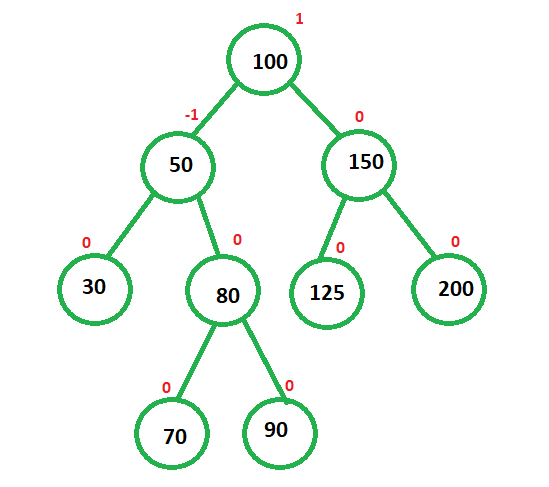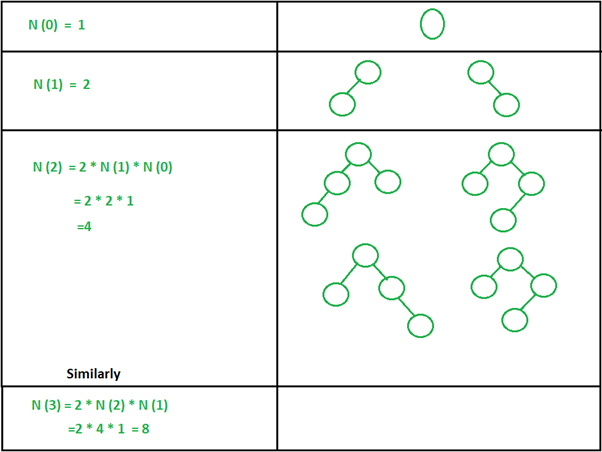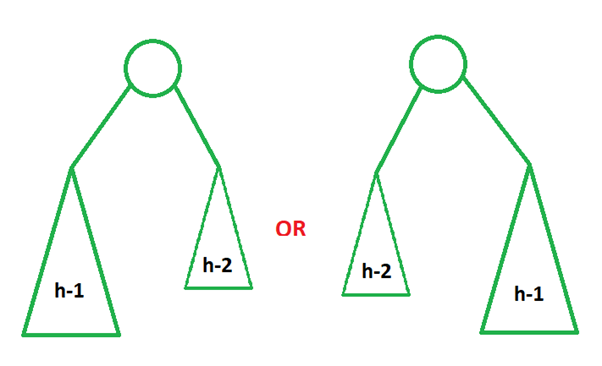GeeksforGeeks App
Open AppBrowser
Continue

# Different shapes of AVL possible at height h

AVL Tree: It is a self-balancing Binary Search Tree where the Balance Factor cannot be more than one for all nodes. Balance Factor can be defined as the difference between heights of left and right subtree.

Example:The task is to find the possible number of different shapes of a minimal AVL tree of height h can be formed. This can be understood by creating trees and then generating a generalized formula for this computation.

Solution:

Let N(h) denotes the number of possibilities of trees and h denotes the height of the AVL tree. In the figure below, possible trees are shaped along with their height. In the first column, height analysis is shown and in the second column, possible trees are shown.Let us now generalize the above observations:The formula can be generalized as:My Personal Notes arrow_drop_up